## SIGNAL PROCESSING Laboratory #6

### Signal filtering in Python

#### M. Kociński, P. Strumiłło, A. Borowska-Terka Medical Electronics Division Institute of Electronics

In :
import funkcje_do_lab6 as f6
f6.hide()

Out:
The raw code for this IPython notebook is by default hidden for easier reading. To toggle on/off the raw code, click here.

PURPOSE:

Getting acquainted with basic properties of Finite Impulse Response (FIR) filters and Infinite Impulse Response (IIR) filters, their design in Python environment and their practical applications.

INTRODUCTION:

In :
f6.fig('introduction.png')

Out: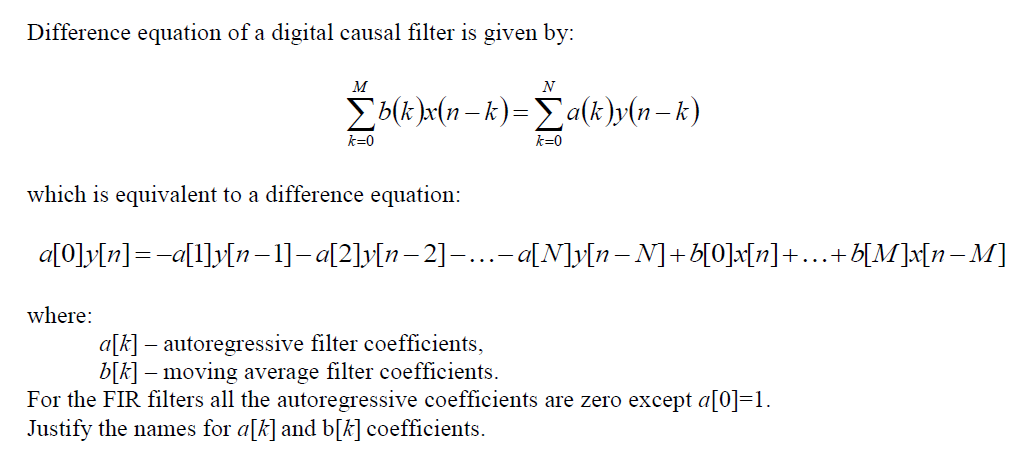Write difference equations and plot block diagrams of the following filters (by default we assume a=1, if a≠1 we need to divide all coefficients values by a ≠0):
b =4, b=2;
b =2, a=-0.5;
b =0.5, a=1;
b =1, b=2, a=0.5, a=-1.

I. FIR filters
Pros:
1) available simple, linear methods for designing filters,
2) linear phase can be easily achieved (symmetry of coefficients),
3) are always stable,
4) feature short transient states (finite impulse response),

Cons: 1) a high number of filter coefficients are required (i.e. high filter order) to obtain steep frequency characteristics.

A FIR filter is given b =0.5, b=0.5. Write a script showing that impulse response of this filter is equal to its moving average coefficients.
Hint: define signal x=[1, 0, 0, ….,0] of length N=100 and sampled at a frequency rate of fs=11400 Hz, and use the lfilter function from the scipy.signal package to obtain the impulse response.

EXPECTED RESULTS:

In :
%pylab inline
pylab.rcParams['figure.figsize'] = (14.0, 7.0)

Populating the interactive namespace from numpy and matplotlib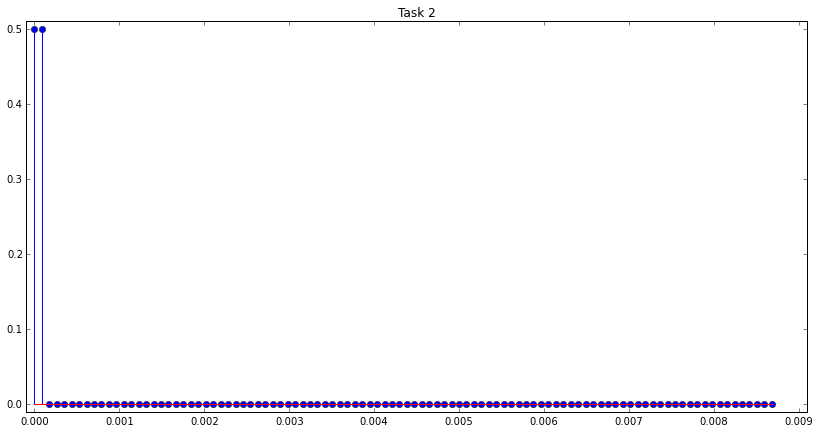Compute frequency characteristic of the filter defined in Task 2 by using the two following methods:
a. by computing the Fourier transform of the impulse response obtained in Task 2; plot the amplitude and phase spectrum of the filter,
b. by using the freqz command from the scipy.signal package. What kind of filter frequency characteristic you have obtained? Is the phase linear? What is the practical consequence of phase linearity?
c. From within the Python environment play the voice_noise.wav wave file (see lab. 3). From the lecture signal database load the wave file voice_noise.wav into the Python workspace (the signal is sampled with a rate of fs=11.4 kHz. Filter the voice signal using the filter from Task 2. Store the filtering result in file voice_filtered.wav. Finally, play the voice_filtered.wav wave file.

In :
f6.task3a()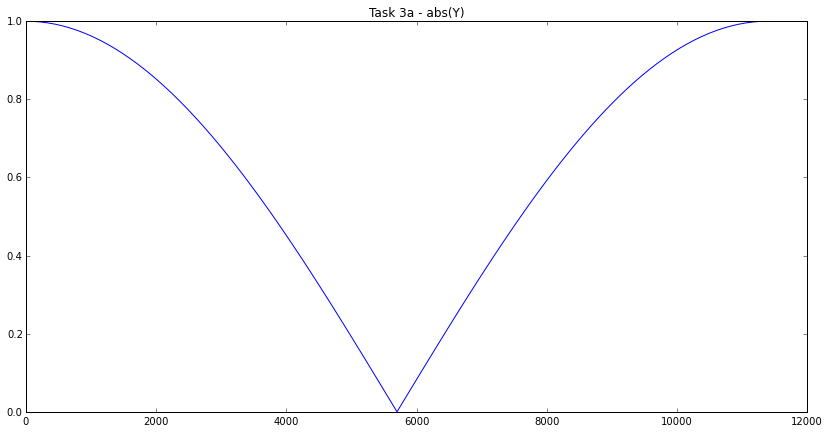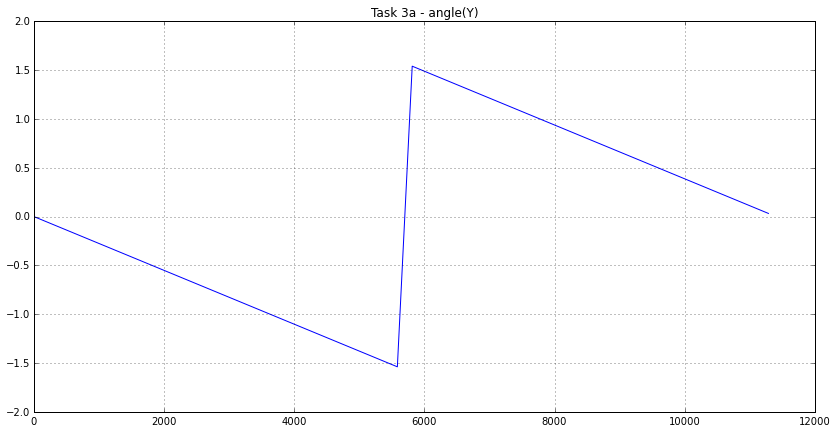In :
f6.task3b()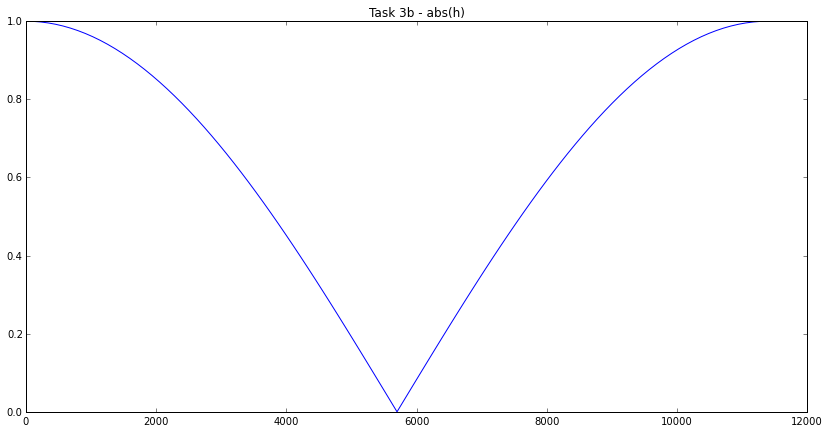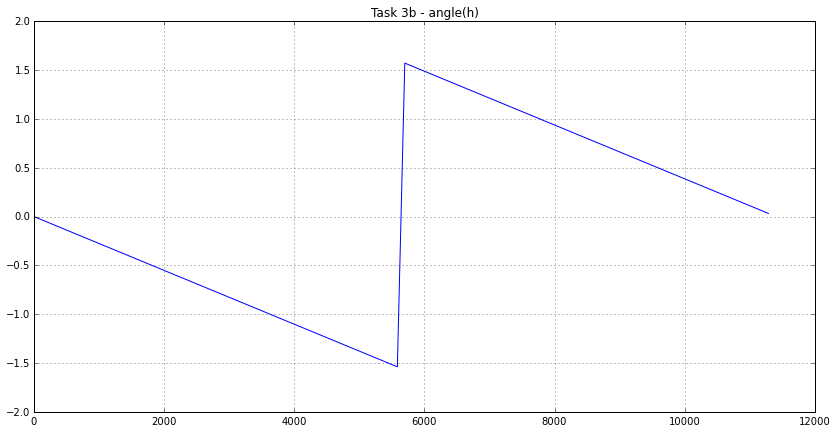The voice_noise.wav wave file:

In :
from IPython.display import Audio
sampling_rate, data=f6.sound(f6.noise_voice)
Audio(data=data, rate=sampling_rate)

Out:
In :
f6.task3c()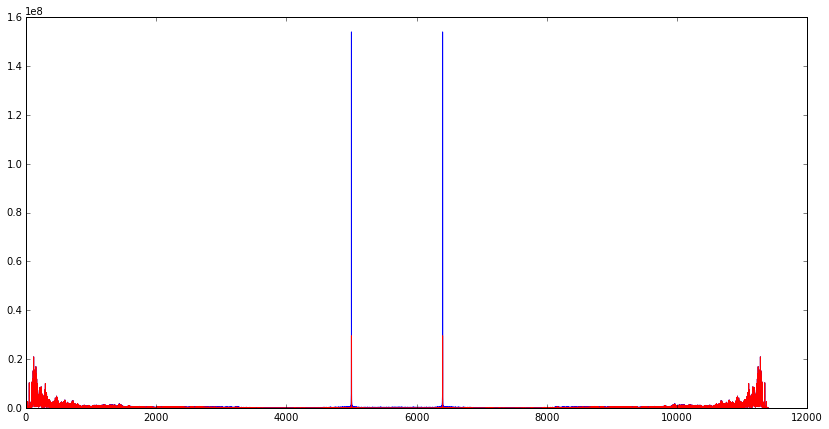The filtered result in file voice_filtered.wav:

In :
from IPython.display import Audio
sampling_rate, data=f6.sound(f6.noise_voice_filtered)
Audio(data=data, rate=sampling_rate)

Out:

Repeat Task 2 for the FIR filter with coefficients b =0.5, b=-0.5.

EXPECTED RESULTS:

In :
f6.task4()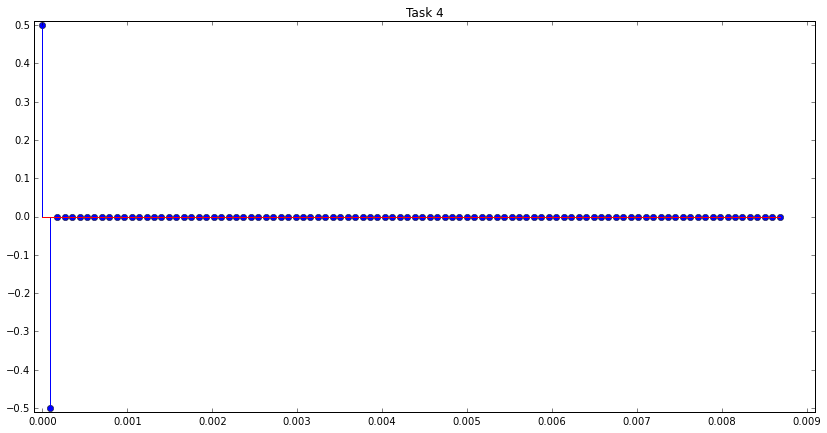Compute the impulse response of a filter which is a cascade connection of the filters studied in Task 2 and Task 4. What is the frequency characteristic of this combined filter.

II. IIR filters
Pros:
- steep frequency characteristics can be achieved for low filter orders.

Cons:
- difficulty in keeping the filter phase linear, - can be unstable.

In :
f6.fig('task6.png')

Out: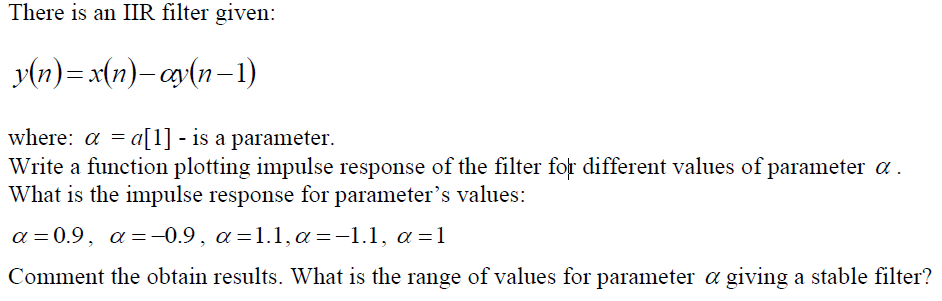EXPECTED RESULTS:

In :
f6.task6()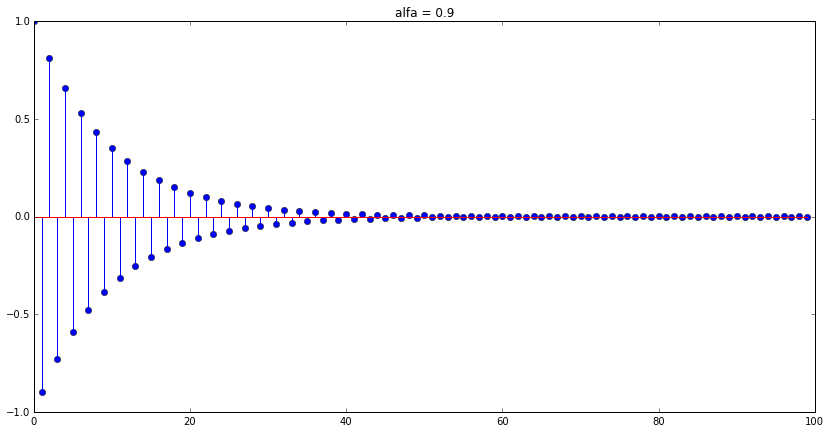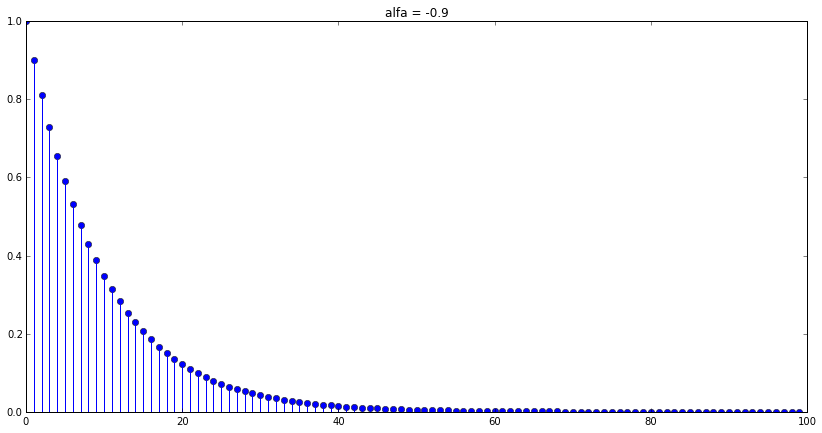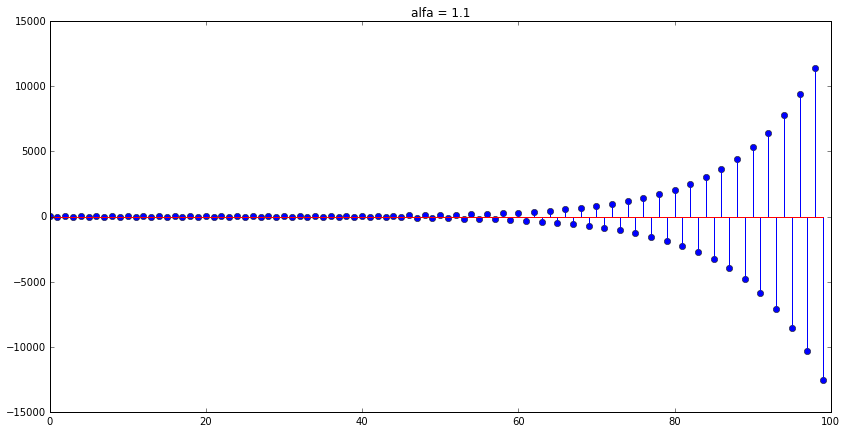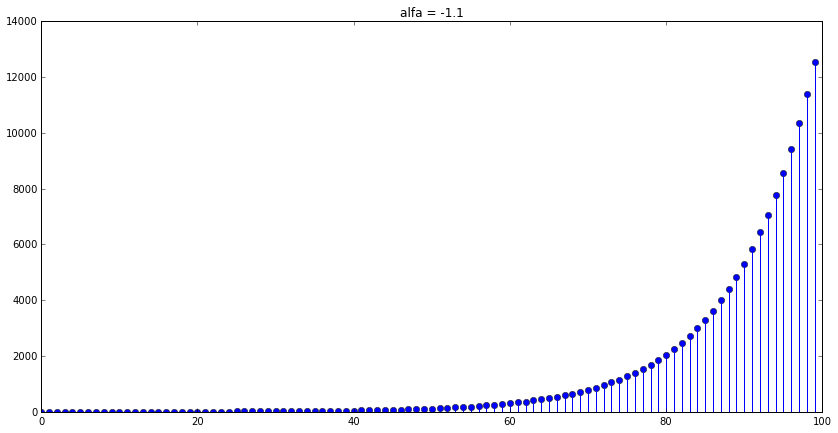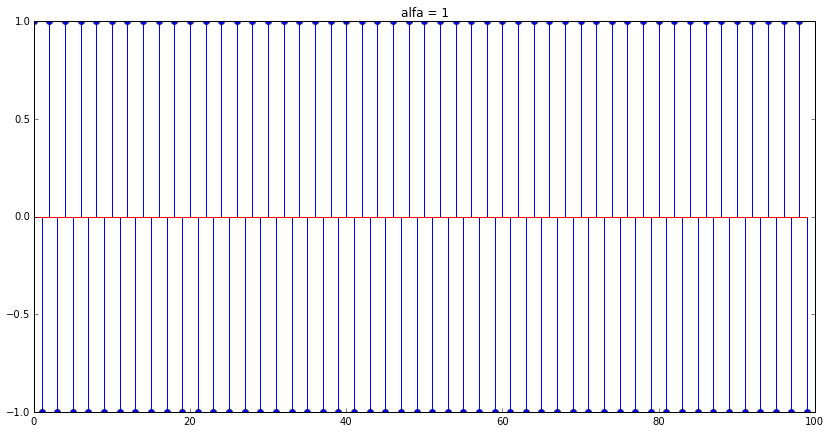Load into the Pylab environment an audio signal male_voice.wav sampled at a rate of fs=11.4kHz. Use the following IIR filter coefficients b=1, a=1, a=-0.7 to filter this signal. Save the result in a wave file. Play the file and comment the obtained audio effect.

The male_voice.wav wave file:

In :
f6.task7()
sampling_rate, data=f6.sound(f6.male_voice)
Audio(data=data, rate=sampling_rate)

Out:

EXPECTED RESULTS:

The filtered result in file male_voice_filtered.wav:

In :
sampling_rate, data=f6.sound(f6.male_voice_filtered)
Audio(data=data, rate=sampling_rate)

Out: